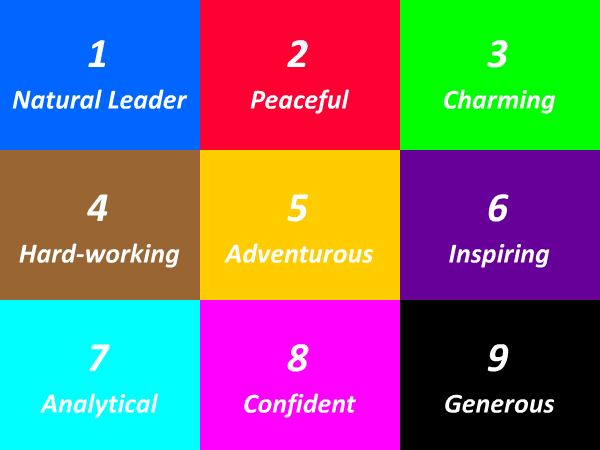# 87 Times Table - Generate Multiplication Table of 87

87 Times Table
1x87=87
2x87=174
3x87=261
4x87=348
5x87=435
6x87=522
7x87=609
8x87=696
9x87=783
10x87=870
11x87=957
12x87=1044
13x87=1131
14x87=1218
15x87=1305
16x87=1392
17x87=1479
18x87=1566
19x87=1653
20x87=1740
21x87=1827
22x87=1914
23x87=2001
24x87=2088
25x87=2175
87 Times Table
26x87=2262
27x87=2349
28x87=2436
29x87=2523
30x87=2610
31x87=2697
32x87=2784
33x87=2871
34x87=2958
35x87=3045
36x87=3132
37x87=3219
38x87=3306
39x87=3393
40x87=3480
41x87=3567
42x87=3654
43x87=3741
44x87=3828
45x87=3915
46x87=4002
47x87=4089
48x87=4176
49x87=4263
50x87=4350
87 Times Table
51x87=4437
52x87=4524
53x87=4611
54x87=4698
55x87=4785
56x87=4872
57x87=4959
58x87=5046
59x87=5133
60x87=5220
61x87=5307
62x87=5394
63x87=5481
64x87=5568
65x87=5655
66x87=5742
67x87=5829
68x87=5916
69x87=6003
70x87=6090
71x87=6177
72x87=6264
73x87=6351
74x87=6438
75x87=6525

## Test Your Skills Here

The 87 times table quiz is very useful in the improvement of kid's multiplication and maths skills. Here you will find a selection of times table tests designed to help your child to learn and practice their times tables. Fill in your answers. Once you have entered all the answers, click on 'Check' button to see whether you have got them all correct. If your answers is Wrong then we will show you the 'Correct' answer.

Practice Excersise
87 x 19 =
87 x 8 =
87 x 14 =
87 x = 174
87 x = 2436
87 x = 2175
87 x = 2610
87 x = 696
87 x = 1305
87 x 30 =#### More Tables#### Top Search Quesries for this page:

• 87 times table
• 87 times tables
• table of 87
• 87 multiplication table
• multiplication chart 87
• multiplication of 87
• 87 table maths### Spreading Knowledge Across the World

USA - United States of America  Canada  United Kingdom  Australia  New Zealand  South America  Brazil  Portugal  Netherland  South Africa  Ethiopia  Zambia  Singapore  Malaysia  India  China  UAE - Saudi Arabia  Qatar  Oman  Kuwait  Bahrain  Dubai  Israil  England  Scotland  Norway  Ireland  Denmark  France  Spain  Poland  and  many more....